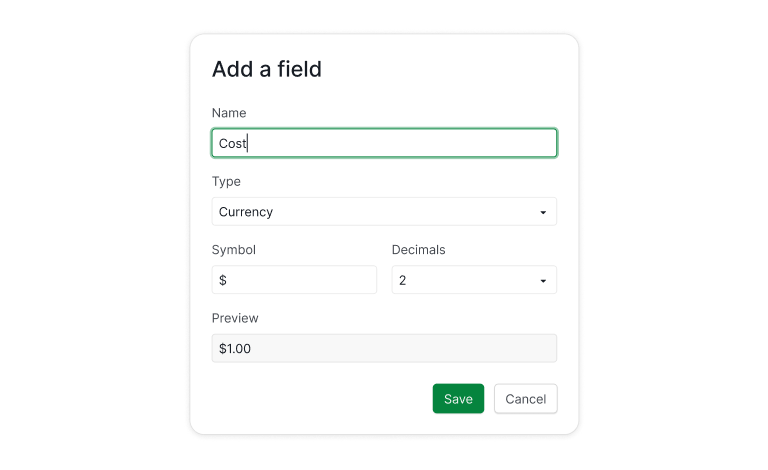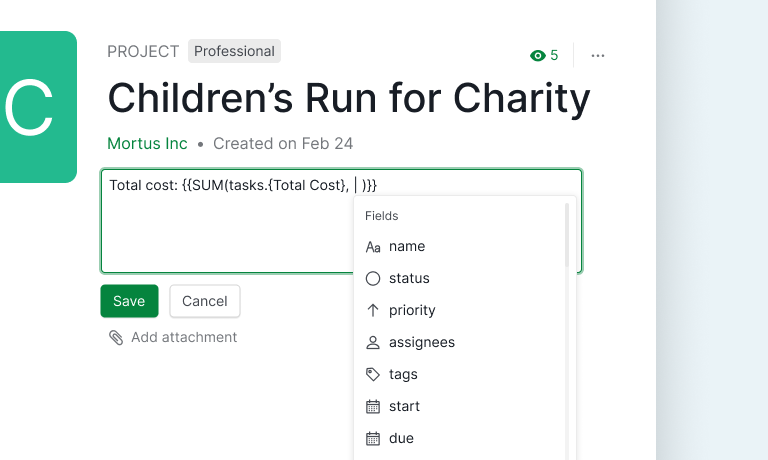## Your Formula for Success: Quire Array FormulasPicture this: you're looking at your project management software, and you're like, "Wait, do array formulas even belong here?" I mean, we usually think of formulas as those things that just add or subtract numbers, right? Well, hold that thought.

Because here's the deal: when you've got a bunch of tasks in a project, each doing its own thing, you need to be the master of metrics to succeed. That’s where array formulas swoop in to save the day. In this blog post, I'm going to show you how these fancy formulas can jazz up your data game. Get ready to dive into your project's world like never before!

Array Formulas is available for all of the subscription tiers. More information can be found on our Pricing page.

# What are Array Formulas?

An array is a collection of data, such as tasks, assignees, durations and numbers. An array formula allows you to perform multiple calculations within the selected collection of data, i.e., an array.

For example, if you’d like to calculate the schedule variant of a task, you can write a formula as follows:

``````timeSpent - estimated
``````

Then, if you would like to calculate the schedule variance of all subtasks, you can write a formula as follows:

``````subtasks.timeSpent - subtasks.estimated
``````

It might look alien to you – I’ll explain it later. Here, you just need to know, in the above formula, `subtasks` is a collection of subtasks, and, with array formulas, you can simply subtract one array from another like manipulating simple values.

You can fiddle how a formula works by simply entering it in the task’s description by enclosing it with `{{` and `}}`. Please visit our guide for more information.

# Some Basic Array Operations that You Should Know

Within Quire’s formula, you can prepare an arbitrary array with `[` and `]`. For example, you can have a collection of 1, 2 and 3 as follows:

``````[1, 2, 3]
``````

Then, if you’d like to multiply with a value, you can do:

``````[1, 2, 3] * 5
``````

The result will be

``````[5, 10, 15]
``````

Also, you can do a calculation for two arrays:

``````[1, 2, 3] - [2, 1, 3]
``````

The result will be

``````[-1, 1, 0]
``````

OK, now we can explain the formula mentioned above now:

``````subtasks.timeSpent - subtasks.estimated
``````

Assuming we have three subtasks, their time spent is 1h, 2h and 3h, and estimated time 2h, 1h, 3h. Then, `subtasks.timeSpent` is actually calculated to `[1h, 2h, 3h]`, while `subtasks.estimated` is calculated to `[2h, 1h, 3h]`. And, the result is `[-1h, 1h, 0h]`.

`1h` means 1 hour duration. Please visit our guide for more information.

# Sorting and Filtering

## The `sort` function

To sort a collection of data, you can simply use the `sort` function:

``````sort([3, 1, 5, 2, 4]) * 2
``````

It will return with the following result:

``````[2, 4, 6, 8, 10]
``````

It is equivalent to `sort(3, 1, 5, 2, 4) * 2`

## The `limit` operator

If you filter only the first three elements, you can use the limit operator as follows:

``````sort([3, 1, 5, 2, 4]) * 2 limit 3
``````

## The `where` operator

If you’d like to filter out smaller values, you can use the where operator as follows:

``````[3, 1, 5, 2, 4] where any > 4
``````

It will return with the following result:

``````[3, 5]
``````

`any` is a keyword to represent each value in the array on the left of the `where` operator.

## The `order by` operator

Let us go back to the formula that calculates the schedule variance and play around with it: `subtasks.timeSpent - subtasks.estimated.`

It returns with the time variance of all subtasks. Now assume we’d like to retrieve the tasks instead of the schedule variance, then we can use the order by operator as follows:

``````subtasks order by any.timeSpent - any.estimated
``````

The default ordering is ascending order. You can change it to descending with the desc keyword as follows:

``````subtasks order by desc any.timeSpent - any.estimated
``````

Here is a more advanced and complicated formula. Let’s try if you can tell what it is up to:

``````subtasks where any.timeSpent > 1d and any.due < tomorrow and any.priority >= high order by desc any.timeSpent - any.estimated limit 3
``````

# Knowing Your Project Dynamics with Array Formulas

## Custom Field with Formulas

Applying this is a piece of cake – just create a custom field with a formula. Let's say you're all about keeping tabs on how much each task is costing you. First of all, you can define a custom field, say, `Cost` to record the cost for each task.Then, you can define another field, for example, `Total Cost` to calculate the total cost of each task including its subtasks as follows.There are a few things worth to pay attention to:

1. The formulas are case insensitive. That is, `Cost` and `cost` are equivalent. So are `SUBTASKS` and `subtasks`.
2. If a field’s name contains spaces or other non- alpha-numeric characters, you have to enclose it with `{` and `}`. It is why you see `{Total Cost}` in the above formula.

Let us dig a bit further about how `Total Cost` is calculated. At first, assume we have three tasks: A, B and C. And, B and C are subtasks of A. Also, you entered `Cost` for A, B, C as 50, 30, 10, respectively. Then,

1. The total cost of B and C will be 30 and 10, since they don’t have any subtasks.
2. A’s total cost will be `SUM(30, 10, 50)`. It will be 90.

## Summarize Project Dynamics in its DescriptionHere's another cool way to use formulas – you can slip them right into the project description. Imagine you want to show the total project cost. Just jot down something like this in the project description:

``````Total cost: {{SUM(tasks.{Total Cost})}}
``````

Where:

1. The description is Quire-flavored markdown. To specify a formula, you have to enclose it with `{{` and `}}`. Also, once entering `{{`, an auto-complete dialog will help you to complete the formula.
2. `tasks` is a built-in identifier to represent all tasks in the project. Again, it is case insensitive.

If we’d like to know the most 5 expensive tasks, we can do:

``````{{tasks order by desc any.{Total Cost} limit 5}}
``````

Or, you’d like to list the tasks that spent too much time:

``````{{tasks where any.timeSpent - any.estimated > 1d order by desc any.timeSpent - any.estimated limit 5}}
``````

You can put the formula into any description, as long as the markdown is supported, including tasks and comments. You can even summarize the performance of your colleagues by writing down the proper formulas in the description of his profile.

To learn and explore how Quire array formulas can help you, you can try it out in a task’s description. It is more convenient for trial-and-error.

# Let’s Write Your First Formula in Quire!

So, there you have it, the scoop on array formulas in Quire – your ultimate project management sidekick. And guess what? Quire isn't just hopping on the array formula train; it's driving the thing! It's the pioneer, the trailblazer – the first-ever project management software to bring you array formulas, and not just that, it's acing the game.

With Quire, array formulas aren't just a feature; they're an experience that's fine-tuned to perfection. So, if you're all about supercharging your project insights, Quire's array formulas are here to rock your world. Get ready to crunch numbers, uncover insights, and take your project management to a whole new level with Quire's cutting-edge array formulas. Your projects will thank you – and so will your organized, data-loving side!